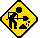Datasheet legend
Ab/c: Fractions calculation
AC: Alternating current
BaseN: Number base calculations
Card: Magnetic card storage
Cmem: Continuous memory
Cond: Conditional execution
Const: Scientific constants
Cplx: Complex number arithmetic
DC: Direct current
Eqlib: Equation library
Exp: Exponential/logarithmic functions
Fin: Financial functions
Grph: Graphing capability
Hyp: Hyperbolic functions
Intg: Numerical integration
Jump: Unconditional jump (GOTO)
Lbl: Program labels
LCD: Liquid Crystal Display
LED: Light-Emitting Diode
Li-ion: Lithium-ion rechargeable battery
Lreg: Linear regression (2-variable statistics)
mA: Milliamperes of current
Mtrx: Matrix support
NiMH: Nickel-metal-hydrite rechargeable battery
Prnt: Printer
RTC: Real-time clock
Sdev: Standard deviation (1-variable statistics)
Solv: Equation solver
Subr: Subroutine call capability
Symb: Symbolic computing
Tape: Magnetic tape storage
Trig: Trigonometric functions
Units: Unit conversions
VAC: Volts AC
VDC: Volts DC
 Years of production: Display type: Graphical display New price: Display color: Black Display technology: Liquid crystal display Size: 7"×3½"×1" Display size: pixels Weight: 10 oz Entry method: Formula entry Batteries: 4×"AAA" alkaline Advanced functions: Trig Exp Hyp Lreg Grph Ab/c Cplx Cmem Mtrx External power: Memory functions: +/- I/O: Programming model: Formula programming Precision: 14 digits Program functions: Jump Cond Subr Lbl Ind Memories: 23064(0) bytes Program display: Formula display Program memory: 23064 bytes Program editing: Formula entry Chipset: Forensic result: 9.000000098906The EL-9300C is a sophisticated graphical calculator from Sharp. What I have here is a prototype overhead version of this machine.```Input x
y=1
Label 1
If x>0Goto 2
y=x*y
x=x+1
Goto 1
Label 2
g=1+(76.18009172+9.5E-9)/(x+1)-86.50532033/(x+2)+
24.01409824/(x+3)-1.231739572/(x+4)+
1.208650973E-3/(x+5)-5.395239384E-6/(x+6)
e^(ln (g*G√(2π)/x)+(x+.5)*ln (x+5.5)-x-5.5)/y```